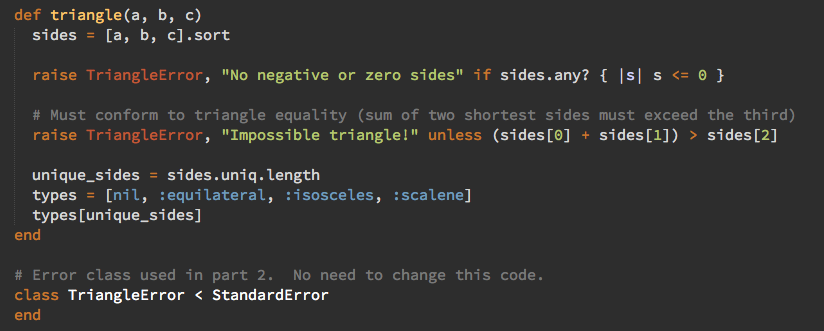# Jamie's Blog

Ruby developer. CTO. Swimmer. Always trying to write more

# The Triangle QuestionI’m currently entertaining myself by working through the Ruby Koans which I’d somehow missed over the last few years.

Most of the questions are fairly mundane like

``````def test_iterating_with_each
array = [1, 2, 3]
sum = 0
array.each do |item|
sum += item
end
assert_equal __, sum
end
``````

but the “Triangle question is a bit of fun. You’re given a test like:

``````class AboutTriangleProject < Neo::Koan
def test_equilateral_triangles_have_equal_sides
assert_equal :equilateral, triangle(2, 2, 2)
assert_equal :equilateral, triangle(10, 10, 10)
end

def test_isosceles_triangles_have_exactly_two_sides_equal
assert_equal :isosceles, triangle(3, 4, 4)
assert_equal :isosceles, triangle(4, 3, 4)
assert_equal :isosceles, triangle(4, 4, 3)
assert_equal :isosceles, triangle(10, 10, 2)
end

def test_scalene_triangles_have_no_equal_sides
assert_equal :scalene, triangle(3, 4, 5)
assert_equal :scalene, triangle(10, 11, 12)
assert_equal :scalene, triangle(5, 4, 2)
end
end
``````

and told to implement the `triangle` method

``````# Triangle Project Code.

# Triangle analyzes the lengths of the sides of a triangle
# (represented by a, b and c) and returns the type of triangle.
#
# It returns:
#   :equilateral  if all sides are equal
#   :isosceles    if exactly 2 sides are equal
#   :scalene      if no sides are equal
#
# The tests for this method can be found in
# and
#
def triangle(a, b, c)
end

# Error class used in part 2.  No need to change this code.
class TriangleError < StandardError
end
``````

I started typing `if a == b…` but quickly realised that there was no branching logic needed at all. All the triangle method needed to do count the number of unique sides so instead of a messy conditional statement like

``````if (a==b and a==c)
value = :equilateral
else
if (a==b or a==c or b==c)
value = :isosceles
else
value = :scalene
end
end
``````

…we can just say:

``````def triangle(a, b, c)
sides = [a, b, c]
unique_sides = sides.uniq.length
types = [nil, :equilateral, :isosceles, :scalene]
types[unique_sides]
end
``````

This uses the number of unique sides as the array index to determine the triangle type. Even adding the error checking required later, can be done without messy conditionals:

``````def triangle(a, b, c)
sides = [a, b, c].sort

raise TriangleError, "No negative or zero length sides" if sides.any? { |s| s <= 0 }

# Must conform to triangle equality (sum of two shortest sides must exceed the third)
raise TriangleError, "Impossible triangle!" unless (sides + sides) > sides

unique_sides = sides.uniq.length
types = [nil, :equilateral, :isosceles, :scalene]
types[unique_sides]
end
``````

I think this would make a good interview question. Not because it’s particularly hard or tricky (interview questions shouldn’t be) but because there are multiple ways to solve it and it encourages a good discussion.

How could we rewrite `if a <= 0 || b <= 0 || c <= 0` using a block? What are the disadvantages of my array indexing approach? How would you rewrite it to use a `case…when` structure?

Note: The Koans are about learning and enlightenment and are not a test. Don’t set them all as interview test — they’re too long, and easy to “cheat” because the test suite will give you the next expected answer. This triangle question could be extracted though. Also, setting up a Nitrous.io instance for your candidate means they don’t have to set anything up on their own machine.